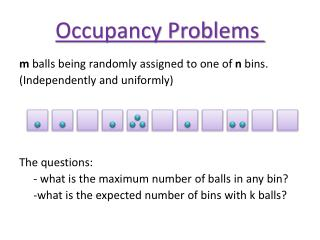DownloadDownload PresentationOccupancy Problems

Occupancy Problems

Télécharger la présentationOccupancy Problems

- - - - - - - - - - - - - - - - - - - - - - - - - - - E N D - - - - - - - - - - - - - - - - - - - - - - - - - - -
Presentation Transcript

1. Occupancy Problems m balls being randomly assigned to one of n bins. (Independently and uniformly) The questions: - what is the maximum number of balls in any bin? -what is the expected number of bins with k balls?

2. For arbitrary events: , not necessarily independent: the probability of the union of events is no more than the sum of their probabilities. Let m=n : For let , where j is the number of balls in the th bin. Then we get: for all i.

3. Now: we concentrate on analyzing the 1st bin, so: Let denote the event that bin has or more balls in it. So: From upper bound for binomial coefficients

4. Now, let Then: with probability at least , no bin has more than balls in it!

5. The Birthday Problem Now n=365, How large must m be before two people in the group are likely to share their birthday? For , let denote the event that the th ball lands in a bin not containing any of the first balls. But:

6. So: Now we can see that for the probability that all m balls land in distinct bins is at most .

7. Markov Inequality Let Y be a random variable assuming only non-negative values. Than for all : Or: Proof:define Than: Now, , , else

8. standard deviation If X is a random variable with expectation , The variance is defined: The standard deviation of X is

9. Chebyshev’s Inequality Let X be a random variable with expectation , and standard deviation . Then for any : Proof: First: for we get . applying the markov inequality to Y bounds this probability.